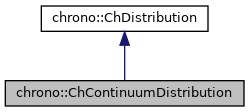chrono::ChContinuumDistribution Class Reference

## Description

Class that can be used to generate sample numbers according to a probability distribution.

Probability distribution is defined with x,y points, at least a dozen of pairs to be more precise in the reconstruction of probability. It uses the "Smirnov transform" (inverse probability integral transform)

#include <ChDistribution.h>

Inheritance diagram for chrono::ChContinuumDistribution:[legend]
Collaboration diagram for chrono::ChContinuumDistribution:[legend]

## Public Member Functions

ChContinuumDistribution (ChVectorDynamic<> &mx, ChVectorDynamic<> &my)
Create an object that can be used to generate sample numbers according to a generic probability distribution. More...

virtual double GetRandom () override
Compute a random value whose probability is the probability curve that has been entered with x,y points during the creation of this object.

const ChVectorDynamicGetProbabilityXpoints () const

const ChVectorDynamicGetProbabilityYpoints () const

const ChVectorDynamicGetProbabilityCDFcumulativeX () const

const ChVectorDynamicGetProbabilityCDFcumulativeY () constPublic Member Functions inherited from chrono::ChDistribution
virtual ~ChDistribution ()
Default destructor for distribution object.

## ◆ ChContinuumDistribution()

 chrono::ChContinuumDistribution::ChContinuumDistribution ( ChVectorDynamic<> & mx, ChVectorDynamic<> & my )

Create an object that can be used to generate sample numbers according to a generic probability distribution.

The probability distribution is a curve represented by simplified x,y pairs of points. The integral of the probability curve must be unit, i.e normalized (but if not, a normalization will be enforced) Note: too few points means approximate results, but too many points might give a small performance overhead when calling GetRandom().

The documentation for this class was generated from the following files:
• /builds/uwsbel/chrono/src/chrono/core/ChDistribution.h
• /builds/uwsbel/chrono/src/chrono/core/ChDistribution.cpp